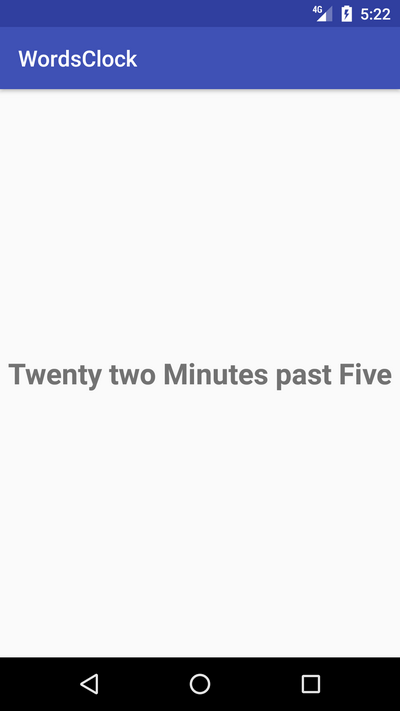In that tutorial, you are going to discover how to display the current time in English words on Android.

As a developer, you know probably how to get the current time via the standard SDK but do you know how to display this time in English words to let the users to read directly the time on the screen ?

Note that you can discover this tutorial directly in video on YouTube :

To display the current time in English Words, we will need to make our own algorithm. But first, we are going to create the user interface (UI) of our Android application.

The UI will be quite simple with a simple TextView centered on the screen :

## Algorithm to convert time to English Words

Now, we are going to create an algorithm to convert the current time in English Words. In our algorithm, we will need an array to store words used to write the time in English.

Then, we must get the current time. From this time, we extract the hour and the minutes values. In the algorithm, we start by checking if we need to print the word minute or minutes. We must print minute if the value of minutes is one or fifty nine.

When we have minutes from thirty one to fifty nine, we print the hour ahead of the given hour like six fifty five will be five minutes to seven and not five minutes to six when we print the hour ahead of the give hour, we face a problem at hour twelve because if we print an hour ahead of twelve, it will be thirteen.

But, we want one so the code will check this and decides what hour to print in words when minutes are from thirty one to fifty nine. If hour is twelve, we store one. Otherwise, we store words an ahead of given hour.

For the rest of the algorithm, we process like that :

• For minutes, we check if minutes are equal to zero then we add the O’Clock value.
• Then, if minutes are equal to fifteen, we add Quarter Past before the words.
• If minutes are equal to thirty , we add Half past before the words.
• If minutes are equal to forty five, we add Quarter to just before.
• If minutes are lower than thirty, so for minutes between one and twenty nine, we create a specific display.
• And finally in the else part, we create the String to return for the minutes between thirty one and fifty nine.

That gives us the following code for our algorithm letting you to get current time in English Words :

## Using the Algorithm in the Application

Now, we can use this algorithm in our Android Application. To refresh the current time continually, we will use a Timer object and we will schedule a TimerTask at fixed rate.

In this TimerTask object, we will get the current time in English Words and we will just have to update the text in the TextView. This gives us the following code for the Main Activity :

The execution of the Android Applications gives us the expected result :That’s all for that tutorial. To discover more tutorials on Android development, don’t hesitate to subscribe to the SSaurel’s Channel on YouTube :